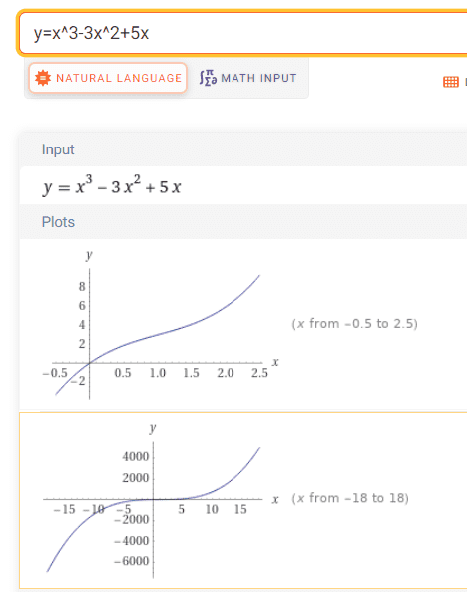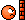# Sum of two real numbers

• POTW
• anemone

#### anemone

Gold Member
MHB
POTW Director
If a and b are two real numbers such that ##a^3-3a^2+5a=1## and ##b^3-3b^2+5b=5##, evaluate a + b.

•bob012345, dextercioby, malawi_glenn and 2 others

There is probably a more elegant solution than mine, but this is what I came up with.
Let ##f(x) = x^3-3x^2+5x##.
Note that ##h(x) = f(x)-3## is antisymmetric around ##x=1##: ##h(1-\xi) = - h(1+\xi)##. For instance ##h(-1) = -12## and ##h(3) = 12##.
To prove this, construct ##g(x) = f(x+1) - 3 = x^3+2x## which is antisymmetric around ##x=0##.
In other words ##g(\xi) + g(-\xi) = 0 \: \forall \xi \in \mathbb{R}##. This means that the polynomial function ##f(x)## satisfy ##f(1-\xi) + f(1+\xi) = 3+ 3 = 6##. Note that ##1-\xi + 1+\xi =2##. Hence, as long as ## f(a) + f(b) = 6## we must have ##a +b= 2##. In the problem, we are given this since ##1+5=6##.

How did I come up with this? I differentiated ##f(x)## and noted that ##f'(x) = 0## has no real solutions. This means means that ##f(x)## must have an antisymmetry property as follows. There exists a constant ##c## s.t. ##h(x) = f(x) -c## is antisymmetric around a certain ##x##-value, say ##x_0##. We thus have ##h(x_0 + \xi) = -h(x_0 - \xi)##.
Then we figure out which ##x##-value provides this antisymmetry.
Set ##f(x+\alpha) + \beta = x^3 + \gamma x##, the RHS is the only valid polynomial which is antisymmetric around ##x=0##.
You will quickly find that ##\alpha = 1##, and then you evaluate ##f(x+1)## to figure out ##\beta## (##\beta = -3##).

•anemone, Wrichik Basu, bob012345 and 1 other person
Subtract polynomials.

Subtract polynomials.
I tried that first came nowhere, perhaps i made some arthimetic error

My solution is probably less elegant but here goes;

Since we are given constraints ##a^3-3a^2+5a=1## and ##b^3 -3b^2 +5b=5##​
we have
$$5a= \left(1- a^3 + 3a^2 \right)$$ likewise $$5b= \left(5- b^3 + 3b^2 \right)$$

$$5(a+b) = 6 - \left (a^3 + b^3\right) +3\left(a^2 + b^2\right)$$

but we know

$$a^2 + b^2 = (a + b)^2 - 2ab$$
and
$$a^3 + b^3 = (a + b)^3 - 3a^2b - 3ab^2$$

substituting we have

$$5(a+b) = 6 -\left((a + b)^3 - 3a^2b - 3ab^2\right) +3\left((a + b)^2 - 2ab\right)$$

expand and regroup

$$5(a+b) = 6 - \left((a + b)^3 - 3ab(a + b) \right) +3\left((a + b)^2 - 2ab\right)$$

let ##z = a+b##

$$5z = 6 - z^3 + 3abz +3z^2 -6ab$$

or

$$5z = 6 - z^3 +3z^2 -3ab(z-2)$$

rearranging

$$z^3 -3z^2 + 5z = 6 - 3ab(z-2)$$

it would sure be convenient if ##z = 2## so we will test that

$$2^3 - 3(2^2) + 5(2) = 6 - 0?$$

$$8 - 12 + 10 =6$$

so ##z= a + b = 2##

[\SPOILER]

Last edited:
•anemone, PeroK, olivermsun and 4 others
$$a+b:=n$$
From the equation of b, we get
$$a^3-(3n-3)a^2+(3n^2-6n+5)a-(n^3-3n^2+5n-5)=0$$Comparing this with the equation of a we get n=2.

In detail by the subtraction
$$(n-2)(3a^2+3na+(n-\frac{1}{2})^2+\frac{11}{4})=0$$
and $$3a^2+3na+(n-\frac{1}{2})^2+\frac{11}{4} >0$$
So n=2.

cf.Last edited:
•anemone and bob012345
@bob012345
I also came to this step:
##3ab(x-2) = 6 - x^3 +3x^2 - 5x##
But I did not have the creativity to set ##x=2## here and see that it works out... so I assumed that I had done something wrongNicely done!

Last edited:
•anemone and bob012345
Isn't this supposed to be for secondary-and-high-school-students?

•PeroK and Wrichik Basu
Isn't this supposed to be for secondary-and-high-school-students?
Maybe very gifted high school students...

•PeroK
So, both ##a## and ##b## are roots of the same third order polynomial. Over the complex we may factor this polynomial ##(x-a)(x-b)(x-c) = 0##. Now, the coefficient of ##x^2## is -3 so the sum ##a+b+c=3## while the constant coefficient ##a b c = 0##. We can conclude ##c=0## giving us ##a+b=3##, an elegant solution to the wrong problem

Maybe very gifted high school students...
Maybe, but I think most of the posters here have a good few years education beyond high school.

•bob012345
Maybe, but I think most of the posters here have a good few years education beyond high school.
I do, and I couldn't get it. Anyhow, at least everyone has hidden the replies. Also, you get rusty if you don't use it. If I could have solved it in a unique way, I would have posted a solution too.

•anemone, bob012345 and malawi_glenn
A slightly different approach.
Let $$f(x) = x^3-3x^2+5x$$and note that$$f(x+y) -f(x) -f(y) = 3xy(x +y-2)$$For non-zero ##x,y## we have $$f(x)+f(y) = f(x+y)$$ iff ##x +y =2##. In which case $$f(x) + f(y) = f(2) = 6$$As ##f## is one to one we have ##f(x) + f(y) = 6## iff ##x +y = 2##.

Last edited:
•anemone and bob012345
Thanks for so many participation to this POTW! I am delighted to see all the correct solutions!

Yes, challenging math problems will be used for high school POTW section because it is our goal to post tricky problems that could stimulate students' creativity, curiosity, self-confidence as well as their desire to explore their own ideas to solve hard math problems.•Greg Bernhardt, ohwilleke and PeroK
I think that progress towards that goal is hindered by posting of solutions/hints by STEM graduates and higher within hours of posting the problem.

My approach is tainted by 40 plus years in engineering. To say it is "less elegant" is a criminal understatement.

We are given
$$a^3-3a^2+5a=1$$
and
$$b^3 -3b^2 +5b=5$$
we can factor out the "a" and have
$$a= \frac {1} {a^2-3a+5}$$
likewise $$b= \frac {5} {b^2-3b+5}$$

I put these in a spreadsheet with "a" in one cell and ##\frac {1} {a^2-3a+5}## in an adjacent cell; a third cell calculates the difference. Then used "goal seek" to vary the cell with "a" until the difference is zero. Similar for the equation of "b". Then I add the two goal seek results to get 1.9995, which is "2" by any reasonable accounting.

I know this is cheating in a math subforum.

[\SPOILER]

I think that progress towards that goal is hindered by posting of solutions/hints by STEM graduates and higher within hours of posting the problem.
Look at the last ~two years powt, 0 or 1 reply starting with "no one answered".
The goal is hindered by something else.

•chwala and erobz
We haven't had a sufficiently talented high school student since @etotheipi went into the ergosphere.

•chwala and malawi_glenn
I think that progress towards that goal is hindered by posting of solutions/hints by STEM graduates and higher within hours of posting the problem.
I interpret these questions as being appropriate for a certain level of education such as high school because that's what the students there have had but having more education usually means a broader scope but doesn't mean one can do that problem any easier beyond that level. Once you know trig you can attempt a tricky trig problem but knowing differential equations or just being older won't necessarily help you do that tricky trig problem.

Also, the kind of curious self motivated students such questions are geared for aren't the type to look at the hints and answers first. I think they want to figure it out themselves.

•erobz
I think the questions might be harder for a larger percentage of secondary/high school students...the goal would rather be set easier but challenging questions that may entice a large number of these students to embrace/look forward to physics forums. My thoughts.

•PeroK